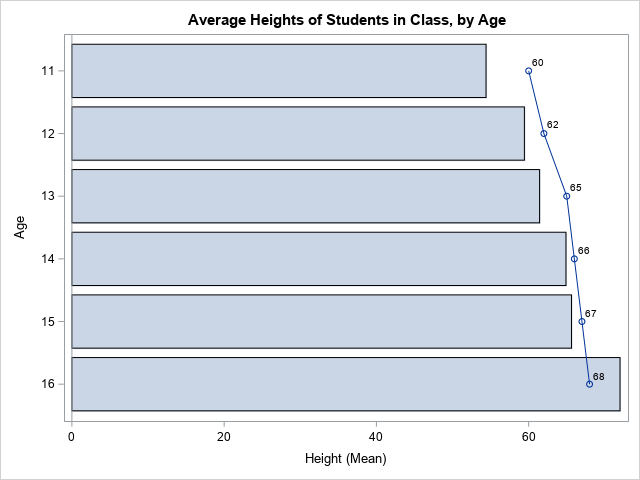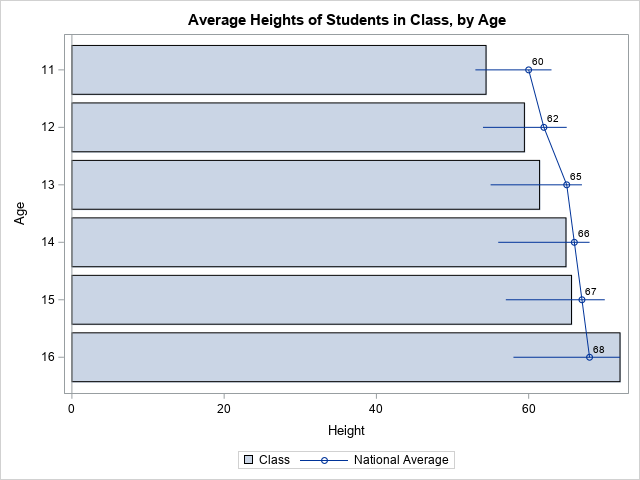It can be frustrating to receive an error message from statistical software. In the early days of the SAS statistical graphics (SG) procedures, an error message that I dreaded was
ERROR: Attempting to overlay incompatible plot or chart types.
This error message appears when you attempt to use PROC SGPLOT to overlay two plots that have different properties. For example, you might be trying to overlay a bar chart, which requires a categorical variable, with a scatter plot or series plot, which often displays values of a continuous variable.

In SAS 9.4M3 and later, there is a simple way to avoid this error message. You can combine a bar chart with other plot types by using the VBARBASIC or HBARBASIC statements, which create a bar chart that is compatible with other "basic" plots.

### Compatibility of plot types

The SAS documentation includes an explanation of chart types, and a table that shows which plots you can overlay when you use PROC SGPLOT or PROC SGPANEL. (The doc erroneously puts HBARBASIC and VBARBASIC in the "categorization" group, but they should be in the "basic group." I have contacted the doc writer to correct the mistake.) If you try to overlay plots from different chart types, you will get the dreaded ERROR: Attempting to overlay incompatible plot or chart types.

First, let me emphasize that this error message only appears when you use PROC SGPLOT or SGPANEL to overlay the plots. If you use the Graph Template Language (GTL) and PROC SGRENDER, you do not have this restriction.

I have previously written about two important cases for which it is necessary to overlay an empirical distribution (bar chart or histogram) and a theoretical distribution, which is visualized by using a scatter plot or series plot:

My previous article shows how to overlay a bar chart and a series plot, but the example is a little complicated. The examples in the next sections are much simpler. There are two ways to combine a bar chart and a line plot: You can use the HBAR and HLINE statements, or you can use the HBARBASIC and SERIES statements. By using HBARBASIC, you can overlay a bar chart with many other plots.

### Overlay a bar chart and a line plot

Suppose you want to use a bar chart to display the average height (by age) of a sample of school children. You also want to add a line that shows the average heights in the population. Because you must use "compatible" plot types, the traditional approach is to combine the HBAR and HLINE statements, as follows. (For simplicity, I omit the legend on this graph.) The DATA step creates fake data, which are supposed to represent the national average and a range of values for the average heights.

```data NationalAverage; /* fake data for demonstration purposes */ label Average = "National Average"; input Age Average Low High; datalines; 11 60 53 63 12 62 54 65 13 65 55 67 14 66 56 68 15 67 57 70 16 68 58 72 ;   data All; set Sashelp.Class NationalAverage; run;   title "Average Heights of Students in Class, by Age"; proc sgplot data=All noautolegend; hbar Age / response=height stat=mean; hline Age / response=Average markers datalabel; run;```This is the "classic" bar chart and line plot. This syntax has been available in SAS since at least SAS 9.2. It enables you to combine multiple statements for discrete variables, such as HBAR/VBAR, HLINE/VLINE, and DOT. However, in some situations, you might need to overlay a bar chart and more complicated plots. In those situations, use the HBARBASIC or VBARBASIC graphs, as shown in the next section.

### Overlay a bar chart and plots of continuous data

The VBARBASIC and HBARBASIC statements (introduced in SAS 9.4M3) enable you to combine bar charts with one or more other "basic" plots such as scatter plots, series plots, and box plots. Like the VBAR and HBAR statements, these statements can summarize raw data. They have almost the same syntax as the VBAR and HBAR statements.

Suppose you want to combine a bar chart, a series plot, and a high-low plot. You can't use the VBAR or HBAR statements because that leads to "incompatible plot or chart types." However, you can use the VBARBASIC and HBARBASIC statements, as follows:

```proc sgplot data=All noautolegend; hbarbasic Age / response=height stat=mean name="S" legendlabel="Class"; series y=Age x=Average / markers datalabel=Average name="Avg" legendlabel="National Average"; highlow y=Age low=Low high=High; keylegend "S" "Avg"; run;```Notice that the SERIES and HIGHLOW statements create "basic" graphs. To overlay these on a bar chart, use the HBARBASIC statement. In a similar way, you can overlay many other graph types on a bar chart.

### Summary

Sometimes you need to overlay a bar chart and another type of graph. If you aren't careful, you might get the error message: ERROR: Attempting to overlay incompatible plot or chart types. In SAS 9.4M3 and later, there is a simple way to avoid this error message. You can use the VBARBASIC or HBARBASIC statements to create a "basic" bar chart that is compatible with other "basic" plots.

ShareDistinguished Researcher in Computational Statistics

Rick Wicklin, PhD, is a distinguished researcher in computational statistics at SAS and is a principal developer of SAS/IML software. His areas of expertise include computational statistics, simulation, statistical graphics, and modern methods in statistical data analysis. Rick is author of the books Statistical Programming with SAS/IML Software and Simulating Data with SAS.

1.Rick,
HBARPARM also can do that .

data NationalAverage; /* fake data for demonstration purposes */
label Average = "National Average";
input Age Average Low High mean;
datalines;
11 60 53 63 60
12 62 54 65 62
13 65 55 67 64
14 66 56 68 63
15 67 57 70 65
16 68 58 72 66
;

data All;
set Sashelp.Class NationalAverage;
run;

proc sgplot data=All noautolegend;
hbarparm category=Age response=mean / name="S" legendlabel="Class";
series y=Age x=Average / markers datalabel=Average name="Avg" legendlabel="National Average";
highlow y=Age low=Low high=High;
keylegend "S" "Avg";
run;

•Correct. If you are using HBARPARM to graph pre-summarized data, you can overlay other "basic" graphs on the bar chart.